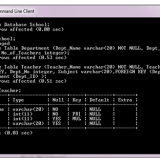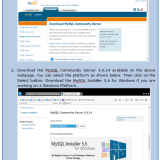Home » Posts tagged "Learn DBMS Online"

## Learn online DBMS “Select Command in MySQL” Complete Lesson 14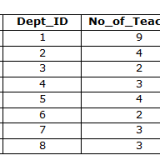SELECT COMMAND–The SELECT Command is used to query a database or retrieve information from it. There are various ways in which the SELECT command can be used. The basic structure of a SELECT command contains the SELECT clause followed by the attribute list (separated by comma (,)) you want to select, then FROM clause followed by the table name and lastly WHERE clause which is followed by the condition which can...

## Learn online DBMS “Delete Command in MySQL” Complete Lesson 13DELETE COMMAND– In order to delete one or more tuples, DELETE command is used. If we want to delete the tuple for Teacher with ID=2345 the command is: DELETE FROM Teacher WHERE Teacher_ID=234;   If the WHERE clause is missing then it will delete all the tuples in a table.      DELETE FROM Teacher;      The above command would delete all the tuple in the table Teacher.        Alike UPDATE...

## Learn online DBMS “Update Command in MySQL” Complete Lesson 12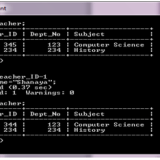UPDATE COMMAND– This command is used to update the attribute values of one or more tuples in a table. For example in the Teacher table, we want to update the Dept_No of teachers teaching computer science to 123. This can be done using the following command: UPDATE Teacher SET Dept_No=123 WHERE Subject=”Computer Science”; We can also use the SELECT command in the WHERE clause of UPDATE command if we have to...

## Learn online DBMS “Insert Command in MySQL” Complete Lesson 11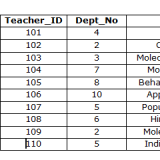INSERT COMMAND – This command is used to insert a tuple in a relation. We must specify the name of the relation in which tuple is to be inserted and the values. The values must be in the same order as specified during the Create Table command. For example, consider the following table Teacher: CREATE TABLE Teacher  ( Teacher_Namevarchar(20) NOT NULL, Teacher_IDinteger, Dept_Nointeger DEFAULT 234,   Subject varchar(20) );  ...

## Learn online DBMS “Alter Table Command” Complete Lesson 10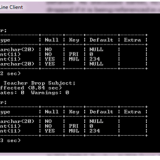ALTER TABLE COMMAND– This command is used to modify the base table definition. The modifications that can be done using this command are: Adding a column: Suppose we want to add a column Date_of_Birth in the Teacher table. Following command is used to add the column: ALTER TABLE Teacher ADD Date_of_Birth date; Teacher table is shown before and after the modification in the following figure: Dropping a column: A column can...

## Learn online DBMS “Drop Table Command in MySQL” Complete Lesson 9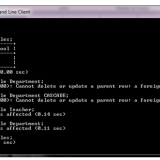DROP TABLE COMMAND:This command is used to delete tables. For example, suppose you want to drop the Teacher table then the command would be:   DROP TABLE Teacher CASCADE;   Thus Teacher table would be dropped and with the CASCADE option, all the constraints that refer this table would also be automatically dropped. However if the requirement is that the table should not be dropped if it is being referenced in...